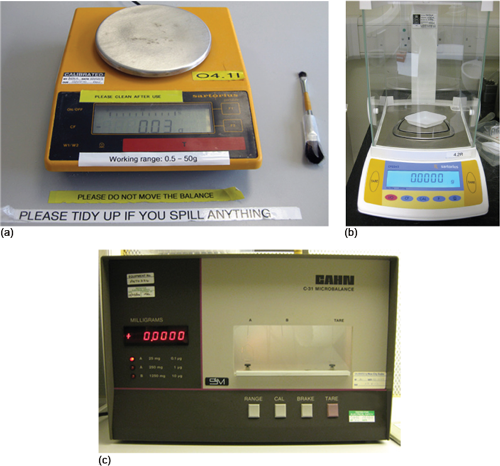Discovering chemistry

Start this free course now. Just create an account and sign in. Enrol and complete the course for a free statement of participation or digital badge if available.

Free course

# 2.1 Weighing solids and liquids in the laboratory

It is probably fair to say that if you made a list of the most frequent operations carried out by a laboratory chemists, weighing samples in one form or other would be at, or at least near, the top.

Examples of different types of laboratory balance are shown in Figure 2.

There are two types of balance: the general-purpose (or top pan) balance (Figure 2a) and the analytical balance (Figure 2b), of which the microbalance (Figure 2c) is a specific type.Figure 2 (a) A general-purpose (or top pan) balance; (b) an analytical balance; (c) a microbalance

Simply looking at the external appearance of both main types reveals a very obvious difference: the analytical balance has an enclosed weighing compartment, the general-purpose balance does not. However, the operational difference lies in the accuracy you require.

General-purpose balances read to two or three decimal places in grams, i.e. their zeroed display will show 0.00 g or 0.000 g respectively. You may come across the terms two-place or three-place used in this context.

However, an analytical balance is far more accurate and reads to four or five decimal places. Microbalances that measure to six decimal places are also quite common (Figure 2c).

• What will be the zero reading on four-place and five-place analytical balances?

• The readings will be 0.0000 g and 0.00000 g respectively.

The correct choice of balance for the job you are required to carry out is essential.

For example, if you were asked to weigh a 5 g sample of a substance to ±0.01g then a general-purpose balance measuring to two decimal places would be ideal. Alternatively, if you were asked to weigh a sample to a much higher degree of accuracy, say 0.5 g of a substance to 0.0001 g, you would need to use an analytical balance measuring to four decimal places.

DC_1